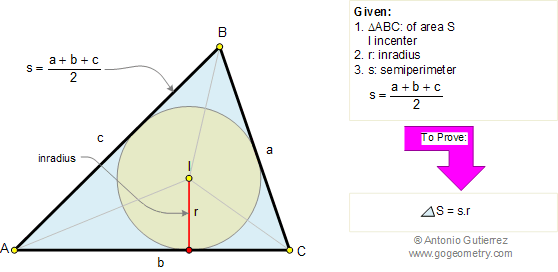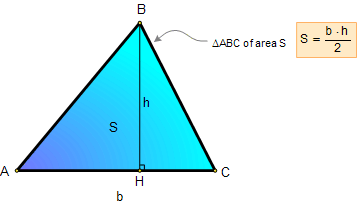Problem 193. Area of a Triangle, Semiperimeter, Inradius
 In the figure below, given a triangle ABC of area S, s is the semiperimeter (a + b + c) / 2, and r is the inradius. Prove that S = s.r View or post a solution1. AREA OF A TRIANGLE:

Proposition: The area of a triangle equals one-half the product of the length of a side and the length of the altitude to that side.Home | Geometry | Search | Problems | 191-200 | Semiperimeter | Area of a Triangle | Email | By Antonio Gutierrez View or post a solution Last updated: Dec 2, 2014